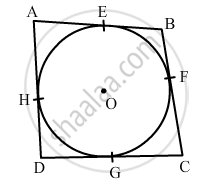Share
Notifications

View all notifications
Books Shortlist
Your shortlist is empty

# A Quadrilateral is Drawn to Circumscribe a Circle. Prove that the Sums of Opposite Sides Are Equal ? - CBSE Class 10 - Mathematics

Login
Create free account

Forgot password?
ConceptCircles Examples and Solutions

#### Question

A quadrilateral is drawn to circumscribe a circle. Prove that the sums of opposite sides are equal ?

#### Solution

Let ABCD be the quadrilateral circumscribing the circle.
Let E, F, G and H be the points of contact of the quadrilateral to the circle.To Prove: AB + DC = AD + BC
Proof:
AB = AE + EB
AD = AH + HD
DC = DG + GC
BC = BF + FC

We have:
AE = AH   (Tangents drawn from an external point to the circle are equal.)
Similarly, we have:
BE = BF
DH = DG
CG = CF
Now, we have:
AB + DC = AE + EB + DG + GC
= AH + BF + DH + CF
= (AH + DH) + (BF + CF)
= AD + BC
⇒ AB + DC = AD + BC

Thus, if a quadrilateral is drawn to circumscribe a circle, the sums of opposite sides are equal.
Hence, proved.

Is there an error in this question or solution?

#### Video TutorialsVIEW ALL 

Solution A Quadrilateral is Drawn to Circumscribe a Circle. Prove that the Sums of Opposite Sides Are Equal ? Concept: Circles Examples and Solutions.
S